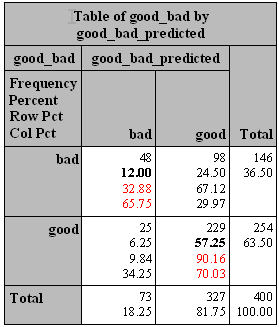1. 这些评估指标，都是可以用白话（plain English, 普通话）解释清楚的；
2. 它们是可以手算出来的，看到各种软件包输出结果，并不是一个无法探究的“黑箱”；
3. 它们是相关的。你了解一个，就很容易了解另外一个。

# 数据

Train data
-------------------------------------------
good        446           74.33

Valid data
--------------------------------------------
good        254           63.50


# 模型

proc logistic data=train;

model good_bad=checking history duration savings property;

run;


Analysis of Maximum Likelihood Estimates

Standard     Wald
Parameter    DF    Estimate       Error     Chi-Square    Pr > ChiSq

Intercept     1      0.6032      0.4466        1.8242        0.1768
checking      1     -0.6536      0.0931       49.3333        <.0001
history       1     -0.4083      0.0980       17.3597        <.0001
duration      1      0.0248     0.00907        7.4820        0.0062
savings       1     -0.2017      0.0745        7.3308        0.0068
property      1      0.3157      0.1052        9.0163        0.0027


logit[p(bad)]=log(p/1-p)
=0.6032-0.6536*checking-0.4083*history+0.0248*duration
-0.2017*savings+0.3157*property


$$p=\frac{exp(logit)}{(exp(logit)+1)}$$

data valid_p;

set valid;

logit=0.6032-0.6536*checking-0.4083*history+0.0248*duration-0.2017*savings+0.3157*property;

p=exp(logit)/(exp(logit)+1);

if p<0.5 then good_bad_predicted='good';

run;


good_bad       p       good_bad_predicted
good      0.12437      good
good      0.21680      good
good      0.34833      good
good      0.48351      good
good      0.03288      good
good      0.06789      good
good      0.15306      good


# Confusion Matrix, 混淆矩阵

1 0
1 d, True Positive c, False Negative c+d, Actual Positive
0 b, False Positive a, True Negative a+b, Actual Negative
b+d, Predicted Positive a+c, Predicted Negative

1. a是正确预测到的负例的数量, True Negative(TN,0->0)
2. b是把负例预测成正例的数量, False Positive(FP, 0->1)
3. c是把正例预测成负例的数量, False Negative(FN, 1->0)
4. d是正确预测到的正例的数量, True Positive(TP, 1->1)
5. a+b是实际上负例的数量，Actual Negative
6. c+d是实际上正例的个数，Actual Positive
7. a+c是预测的负例个数，Predicted Negative
8. b+d是预测的正例个数，Predicted Positive

proc freq data=valid_p;
run;


预测
1	0

b+d, Predicted Positive,73	a+c, Predicted Negative,327	400


### 1. 准确（分类）率VS.误分类率

Accuracy=true positive and true negative/total cases= a+d/a+b+c+d=(48+229)/(48+98+25+229)=69.25%

Error rate=false positive and false negative/total cases=b+c/a+b+c+d=1-Accuracy=30.75%

## 2. （正例的）覆盖率VS. （正例的）命中率

Recall(True Positive Rate，or Sensitivity)=true positive/total actual positive=d/c+d=48/(48+98)=32.88%

Precision(Positive Predicted Value,PV+)=true positive/ total predicted positive=d/b+d=48/(48+25)=65.75%

## 3.Specificity VS. PV-

Specificity(True Negative Rate)=true negative/total actual negative=a/a+b=229/(25+229)=90.16%

Negative predicted value(PV-)=true negative/total predicted negative=a/a+c=229/(98+229)=70.03%

proc freq data=valid_p;

run;proc logistic data=train;

model good_bad=checking history duration savings property;

score data=valid outroc=valid_roc;

run;


• PROB：阈值，比如以上我们选定的0.5
• SENSIT：sensitivity（覆盖率，true positive rate）
• 1MSPEC：1-Specificity，为什么提供1-Specificity而不是Specificity，下文有讲究。
_PROB_ _SENSIT_ _1MSPEC_

0.54866 0.26712 0.07087

0.54390 0.27397 0.07874

0.53939 0.28767 0.08661

0.52937 0.30137 0.09055

0.51633 0.31507 0.09449

0.50583 0.32877 0.09843

0.48368 0.36301 0.10236

0.47445 0.36986 0.10630


# 参考资料：

1. Mithat Gonen. 2007. Analyzing Receiver Operating Characteristic Curves with SAS. Cary, NC: SAS Institute Inc.
2. Dan Kelly, etc. 2007. Predictive Modeling Using Logistic Regression Course Notes. Cary, NC: SAS Institute Inc.
3. Confusion Matrix